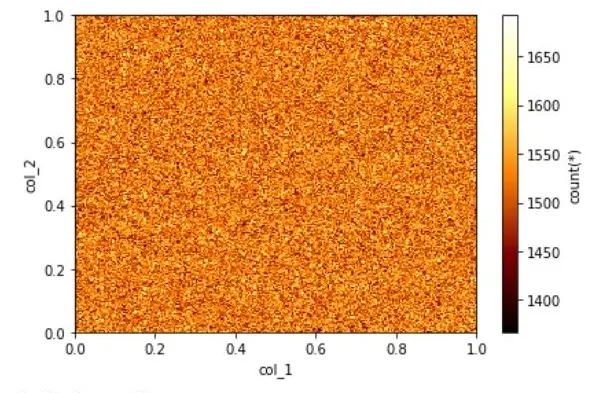# How To Use Vaex To Quickly Analyze 100g Large Amounts Of Data To Break Through The Limitations Of Pandas

### 1. Limitations Of Processing Big Data With Pandas.

1. The Python pandas module is a commonly used data processing tool that can cope with large data sets (tens of millions of rows).
2. But when the amount of data reaches the level of billions of billions of rows, pandas can not process the data set quickly, and the processing speed is very slow.
3. There are performance factors such as computer memory, but panda’s own data processing mechanism (memory dependence) also limits its ability to handle big data.
4. Of course, pandas can read data in batches through chunks, but the disadvantage is that the data processing is more complex, and each step of analysis will consume memory and time.
5. The following example uses pandas to read 3.7 g data sets (HDF5 format), which have 4 columns and 100 million rows and calculate the average value of the first row.
```import pandas as pd

6. When I run this example on my machine, it takes 15 seconds to load the data and 3.5 seconds to calculate the average value, a total of 18.5 seconds.
7. This example uses hdf5 format files. hdf5 is a file storage format. Compared with csv, it is more suitable for storing large amounts of data, with high compression, and faster reading and writing.
8. If you use python’s vaex module to read the same data and do the same average calculation, how much time will it take?
```import vaex

vaex.open('example.h5')```
9. The file reading took 9ms, which can be ignored. The average calculation took 1s, a total of 1s.
10. Why does it take more than ten seconds for pandas to read 100 million rows of HDFS data set, while the time for python vaex is close to zero?
11. This is mainly because pandas read the data into memory and then use it for processing and calculation. But vaex only maps data in memory not read data into memory. This is the same as the lazy load of spark. It will be loaded when it is used. It will not be loaded when declared.
12. So no matter how much data is loaded, 10GB, 100GB… For vaex is instant. The drawback is that vaex only supports HDF5, Apache Arrow, Parquet, FITS, but does not support CSV and other text files, because text files cannot be mapped in memory.

### 2. What Is Vaex?

1. Vaex is also a third-party data processing library based on python, which can be installed with pip.
```\$ pip install vaex

\$ pip show vaex
Name: vaex
Version: 4.1.0
Summary: Out-of-Core DataFrames to visualize and explore big tabular datasets
Home-page: https://www.github.com/vaexio/vaex
Author: Maarten A. Breddels
Author-email: [email protected]
Location: /Library/Frameworks/Python.framework/Versions/3.7/lib/python3.7/site-packages
Requires: vaex-hdf5, vaex-core, vaex-ml, vaex-server, vaex-viz, vaex-astro, vaex-jupyter
Required-by:
```
2. Vaex is a data table tool for processing and displaying data, similar to pandas.
3. Vaex adopts memory mapping and lazy computing, which does not occupy memory and is suitable for big data processing.
4. Vaex can perform second-level statistical analysis and visual display on 10 billion level data sets.

1. Performance: processing massive data, [Formula] rows / sec.
2. Lazy: fast calculation, no memory occupation.
3. Zero memory replication: in filtering/conversion/calculation, the memory is not copied, and the streaming transmission is carried out when necessary.
4. Visualization: including visualization components.
5. API: similar to panda, with rich data processing and calculation functions.
6. Interactive: with jupyter notebook, flexible interactive visualization.

### 4. How To Use Vaex?

1. Vaex supports reading HDF5, CSV, Parquet… format files using the read method. HDF5 can read lazily, while CSV can only read into memory.
```%%time

df = vaex.open('example.hdf5')

Wall time: 13 ms```

#### 4.2 Data Processing.

1. Sometimes we need to do all kinds of data conversion, filtering, calculation and so on. Each step of pandas processing will consume memory, and the time cost is high.
2. Vaex use zero memory in the whole process. Because its processing process only produces expressions, which are logical representations and will not be executed. They will only be executed at the final stage of generating results. And the entire process data is streamed, so there will be no memory backlog.
```%%time
# Filter data, which is an expression and does not consume memory
df_1 = df_v(df_v.col_1>0.5)
Wall time: 142 ms

%%time
# Add the first column and the second column, and then find the maximum value of each row. This is also an expression, which does not consume memory
result = (df_1.col_1 + df_1.col_2).max
Wall time: 0 ms

%% time
# Generate results
result
```
3. As you can see, there are two processes, filtering and calculation, without copying the memory. Here, we use the lazy mechanism of delayed calculation.
4. If each process is calculated in real terms, the time cost will be very large, not to mention the memory consumption.

#### 4.3 Visual Display.

1. Vaex can also be used for rapid visualization display, even if it is tens of billions of data sets, it can still produce graphs in seconds.
```%%time
df_v.plot(df_v.col_1, df_v.col_2, show=True)

Wall time: 573 ms```### 5. Conclusion.

1. Vaex is similar to the combination of spark and panda. The larger the amount of data, the more advantages it has. As long as your hard disk can hold how much data, it can quickly analyze the data.
2. Vaex is still developing rapidly, integrating more and more functions of pandas. Its star number on GitHub is 5K, which has great growth potential.
3. Don’t use pandas to generate HDF5 format files directly, its format will be incompatible with vaex.

This site uses Akismet to reduce spam. Learn how your comment data is processed.